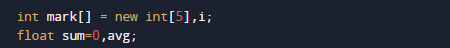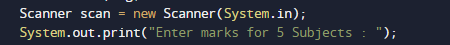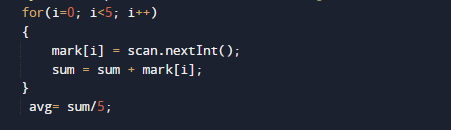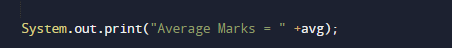# Java Program to Calculate Average Marks

In this tutorial you will learn about the Java Program to Calculate Average Marks and its application with practical example.

In this tutorial, we will learn to create a Java Program to Calculate Average marks  using Java programming.

## Prerequisites

Before starting with this tutorial we assume that you are best aware of the following Java programming topics:

• Java Operators.
• Basic Input and Output function in Java.
• Class and Object in Java.
• Basic Java programming.
• If-else statements in Java.
• For loop in Java.

## Average

Average can be find by adding the numbers(let say we have 5 number then average is “1+2+3+4+5/5”),and then dividing the total by the total number size (5).

In mathematics, an average is called a “mean” = (sum of values)/number of items.

## Java Program to Calculate Average Marks

In our program we will create a program that will Calculate Average of Marks. Firstly we declare required variable and also initiates required values in variable. Next we take values from user at run time and then after we will find the average of Marks.

## OutputIn the above program, we have first declared and initialized a set variables required in the program.

• mark= it will hold entered numbers.
• sum = it will hold sum of values.
• avg = it will hold average of numbers.After that we will takes values from user in variable mark[].And after we will add values taken from user sum=sum+mark[i] and find the average of marks in avg (sum/avg) variable as shown in image below.And finally we print the result i.e sum of all values and average of given values. The above Program is compile and executed, it will produce the following output:In this tutorial we have learn about the Java Program to Calculate Average Marks and its application with practical example. I hope you will like this tutorial.